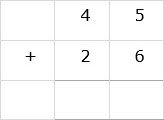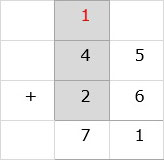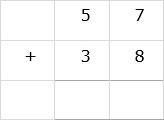# Addition of Two 2-digit Numbers With Carry

Add the numbers 45 + 26

### Solution

Step 1:

We line up the numbers vertically.Step 2:

We add digits in ones column. 5 + 6 = 11. 1 is written below ones column and other 1 is carried over to the tens column.Step 3:

In the tens column, 4 + 2 + 1 = 7. 7 is written below tens column and the final sum is 71.### Solution

Step 1:

We line up the numbers vertically.Step 2:

We add digits in ones column. 7 + 8 = 15. 5 is written below ones column and other 1 is carried over to the tens column.Step 3:

In the tens column, 5 + 3 + 1 = 9. 9 is written below tens column and the final sum is 95.